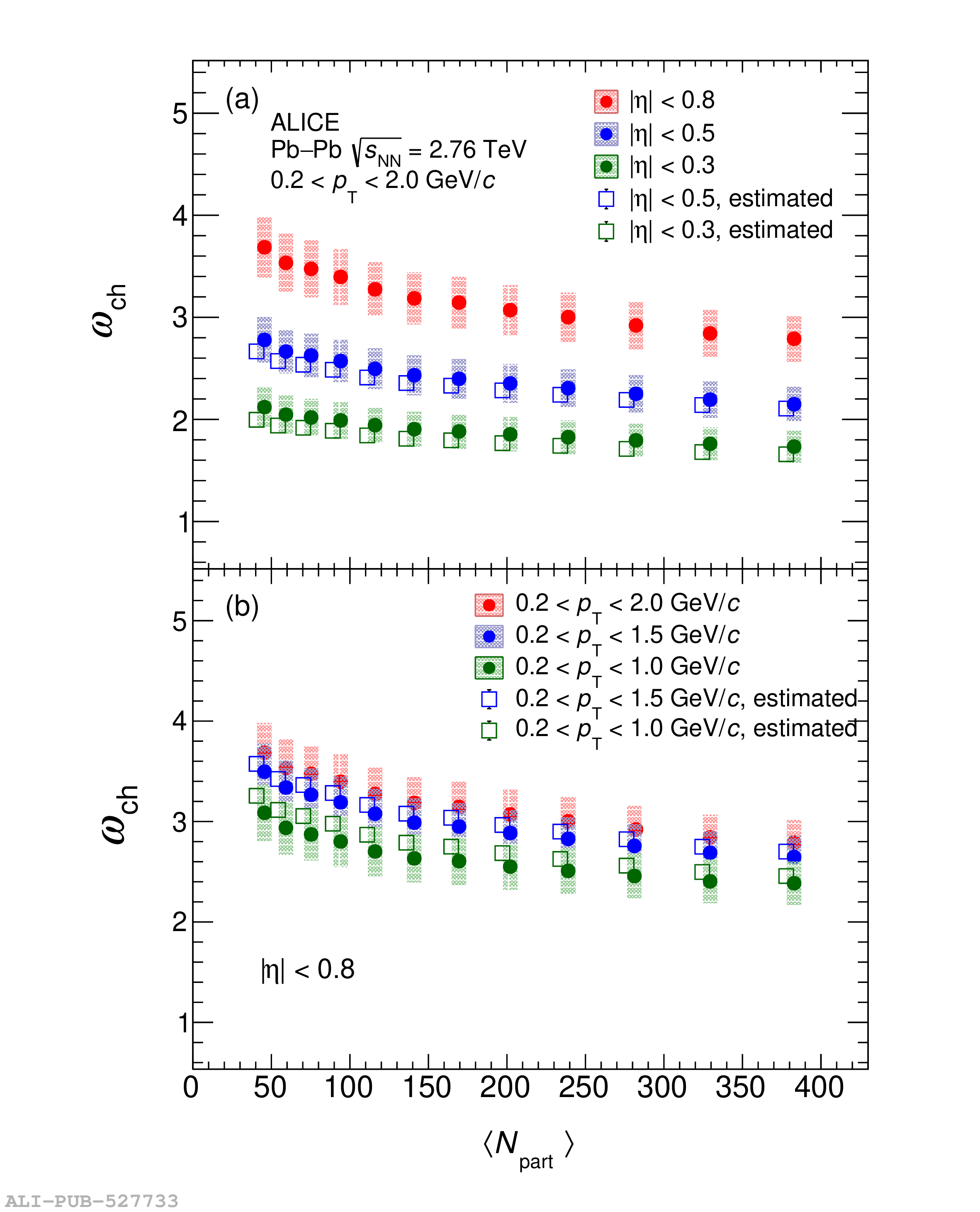# Figure 2

 Scaled variances of charged-particle multiplicity distributions for different $\eta$ and \pT ranges as a function of number of participating nucleons measured in Pb--Pb collisions at \snn $=$ 2.76 TeV, shown inpanels (a), and (b), respectively The estimated $\omega_{\rm ch}$ for $|\eta|<0.3$ and $|\eta|<0.5$ are obtained from the experimental data of $|\eta|<0.8$ by using Eq. \ref {empirical}. The estimated $\omega_{\rm ch}$ for $0.2 < p_{\rm T} < 1.5$ GeV/$c$ and $0.2 < p_{\rm T} < 1.0$ GeV/$c$ are obtained from the experimental data of $0.2 < p_{\rm T} < 2.0$ GeV/$c$, also by using Eq. \ref {empirical}. The statistical uncertainties are smaller than the size of the markers. The systematic uncertainties are presented as filled boxes.